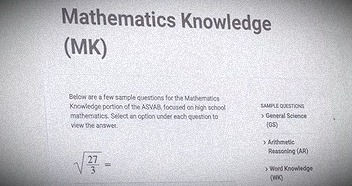top of page
Search

# Mathematics Knowledge of ASVAB testASVAB mathematics knowledge is the second part of the Mathematics section. In this part higher order Mathematics skills of the candidate are test as compared to word problems in Arithmetic reasoning. There are two types of the test like one is computerized and the other is paper and pencil based. In computerized ASVAB Mathematics knowledge test there are a total of 15 questions and the allowed time is 23 minutes. On the other hand, paper and pen type of test contain 25 questions and the allowed time is 24 minutes. Both tests have similar types of questions. Calculators and other electronics gadgets are not allowed in the exam, so don't bring it with yourselves.

Here we will provide you the assistance in different types of questions related to Mathematics Knowledge. It is important to solve as many practice questions as you can, because this will help in understanding the concepts deeply. On the demand of the students some of the questions are solved as follow:

The first question is about simplifying a factor which is under root 27/3. In the below video it is solved and explained as well.

Now moving to the second example which is about finding the volume of the brick. All the parameters of the brick are given therefore the formula of volume will be used to solve the question. The example is solved and explained in the video below.